﻿ 1 μm波段零色散高非线性光子晶体光纤的设计 Design of Photonic Crystal Fiber with Zero Dispersion and High Nonlinearity at the Wavelength of 1 μm

Optoelectronics
Vol. 09  No. 01 ( 2019 ), Article ID: 29450 , 7 pages
10.12677/OE.2019.91011

Design of Photonic Crystal Fiber with Zero Dispersion and High Nonlinearity at the Wavelength of 1 μm

Ningbo Sui, Tiancong Xu, Guosheng Wei, Jinke Li, Zhao Chen

Beijing Glass Research Institute, Beijing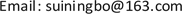Received: Mar. 7th, 2019; accepted: Mar. 20th, 2019; published: Mar. 27th, 2019ABSTRACT

A kind of zero dispersion and high nonlinearity photonic crystal fiber at the wavelength of 1 μm is designed. Characteristics such as dispersion, effective mode area, and nonlinear coefficient of the fiber are investigated by the finite element method. Simulation results show that the dispersion is 0 at the wavelength of 1 μm when the air hole pitch is 4.344 μm, the effective mode area is 11.07 μm2 and the nonlinear coefficient is 18.17 W−1∙km−1. It could be widely used in the high speed optical communications, optic fiber sensors and nonlinear optics.

Keywords:Zero Dispersion, Nonlinearity, Photonic Crystal Fiber, Optic Fiber Sensors

1 μm波段零色散高非线性光子晶体光纤的设计1. 引言

2. 理论分析与光纤结构

$\nabla ×\left[\frac{1}{{\mu }_{r}}\nabla ×E\right]={\left(\frac{\omega }{c}\right)}^{2}{\epsilon }_{r}×E$ (1)

$D=-\frac{\lambda }{c}\frac{{d}^{2}\mathrm{Re}\left({n}_{eff}\right)}{d{\lambda }^{2}}$ (2)

$\gamma =\frac{2\pi {n}_{2}}{\lambda {A}_{eff}}$ (3)

${A}_{eff}=\frac{{\left(\iint {E}^{2}\text{d}x\text{d}y\right)}^{2}}{\iint {E}^{4}\text{d}x\text{d}y}$ (4)

${n}^{2}-1=\frac{0.6961663{\lambda }^{2}}{{\lambda }^{2}-{0.0684043}^{2}}+\frac{0.4079426{\lambda }^{2}}{{\lambda }^{2}-{0.1162414}^{2}}+\frac{0.8974794{\lambda }^{2}}{{\lambda }^{2}-{9.896161}^{2}}$ (5)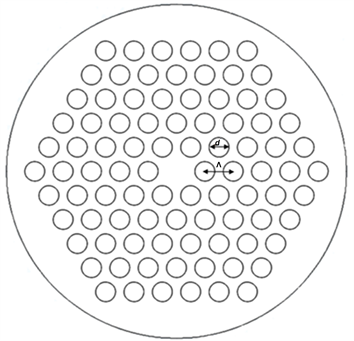Figure 1. Schematic of the PCF

3. 数值模拟和结果分析

3.1. 色散特性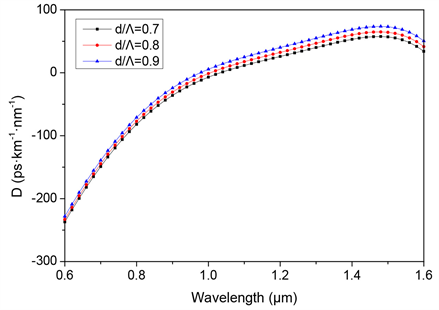Figure 2. D change with wavelength (Λ = 4 μm)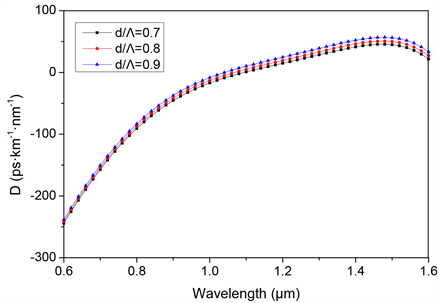Figure 3. D change with wavelength (Λ = 5 μm)Figure 4. D change with wavelength (d/Λ = 0.9)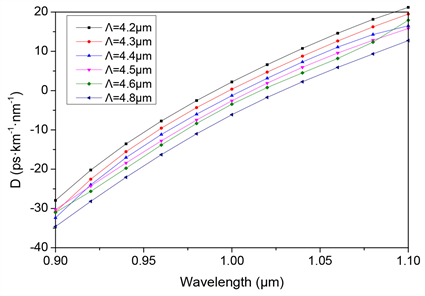Figure 5. D change with wavelength under different Λ

3.2. 有效模场面积及非线性特性

4. 结论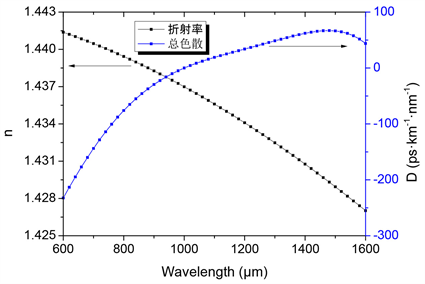Figure 6. neff and D change with wavelength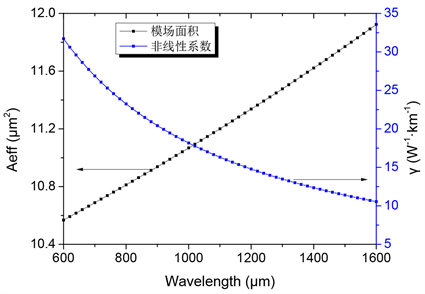Figure 7. Aeff and γ change with wavelength

Design of Photonic Crystal Fiber with Zero Dispersion and High Nonlinearity at the Wavelength of 1 μm[J]. 光电子, 2019, 09(01): 69-75. https://doi.org/10.12677/OE.2019.91011

1. 1. Koeford, J.G., Friis, S.M.M., Christensen, J.B., et al. (2017) Spectrally Pure Heralded Single Photons by Spontaneous Four-Wave Mixing in a Fiber: Reducing Impact of Dispersion Fluctuations. Optics Express, 25, 20835-20849.

2. 2. 王振威, 张晓娟. 反常色散光子晶体光纤中超连续谱的产生[J]. 电脑知识与技术, 2016, 12(33): 254-255.

3. 3. 杨建菊, 韩颖, 王伟, 等. 高非线性光子晶体光纤深紫外超连续谱的研究[J]. 光谱学与光谱分析, 2017, 37(4): 1215-1219.

4. 4. Wang, W., Hou, L.-T., Lu, M., et al. (2009) Design of Double Cladding Nearly Zero Dispersion Flattened Nonlinear Photonic Crystal Fiber. Chinese Physics Letters, 26, 114205-114209.

5. 5. 王二垒, 姜海明, 谢康, 等. 一种高双折射高非线性多零色散波长光子晶体光纤[J]. 物理学报, 2014, 63(13): 134210.

6. 6. 于程程, 励强华, 谷芊志, 等. 一种高双折射光子晶体光纤的特性研究[J]. 哈尔滨师范大学自然科学学报, 2018, 34(2): 82-86.

7. 7. 刘旭安, 吴根柱, 陈达如, 等. 基于椭圆孔包层和微型双孔纤芯的新型高双折射光子晶体光纤[J]. 光子学报, 2011, 40(11): 1728-1732.